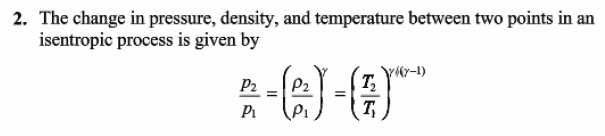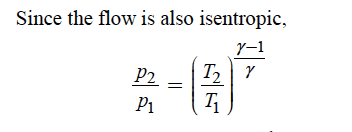# Isentropic process (temperature and pressure relationship)

Gold Member

## Homework Statement

for isentropic process, which of the following is true about the relationship of temperature and pressure?

(1): P2/P1=(T2/T1)^[γ/(γ-1)]
(2): P2/P1=(T2/T1)^[(γ-1)/γ]

where γ = 1.4 for air

stated above

## The Attempt at a Solution

I have found equation (1) in my module lecture notes and both equation (1) and (2) in my textbook.

Let me attached the image of the related part of the textbook here:
For (1): https://i.imgur.com/Z7vPTWX.pngFor (2): https://i.imgur.com/nsrmUL0.pngI feel like they are giving out different answer and I am not sure which one is true or which implies in what specific condition.
Thank you very much!

Last edited:

## Answers and Replies

kuruman
Science Advisor
Homework Helper
Gold Member
Perhaps you can derive the correct expression yourself. Hint: Use the ideal gas law to eliminate the pressure in the isentropic expression pVγ = constant.

•Chestermiller
Gold Member
I remember our lecturer had derived the equation during lecture and what she got is this.
(1): P2/P1=(T2/T1)^[γ/(γ-1)]

Do you think the other equation (i.e. P2/P1=(T2/T1)^[(γ-1)/γ] ) is wrong?
Thanks.

kuruman
Science Advisor
Homework Helper
Gold Member
I think that one of the two equations is a typo. Can you figure out which one? Just reconstruct your lecturer's derivation as I outlined in post #2.

•Chestermiller